×

Search anything:

# Doubly linked list in JavaScript

#### JavaScript linked list Data StructuresIn this article at OpenGenus, I will explain what are doubly linked lists and how to implement them using JavaScript.

1. Singly vs Doubly Linked List
2. Methods:
• append
• prepend
• insertAtIndex
• removeFirst
• removeLast
• removeAtIndex
• getValueAtIndex
3. Summary
4. Complete JavaScript Implementation

## 1. Singly vs Doubly Linked List

Linked lists are data structures, they consists of nodes (collections of data) where each node is linked to each other. The first node is called the Head and the last node is called the Tail, if there is only one node in the list then that is both the Head and Tail.

There's a little difference between singly and doubly linked lists.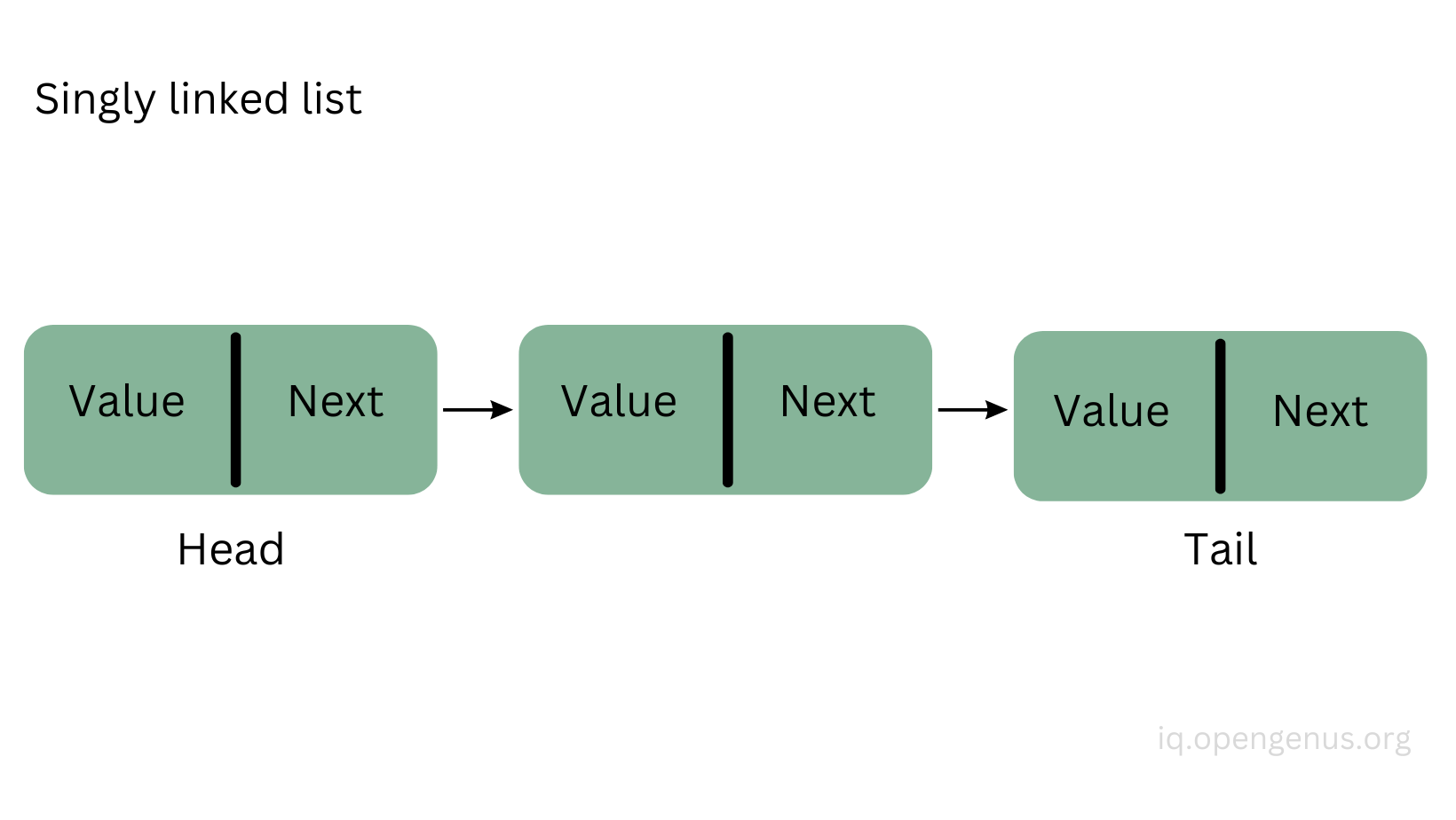Singly linked lists have a value and a next property. The next property will point to the next node in the list and the tail's next property (tail.next => null) will point to null because there's no more node after it. It's much easier to work with singly linked lists because to traverse the list, it only happens in one direction.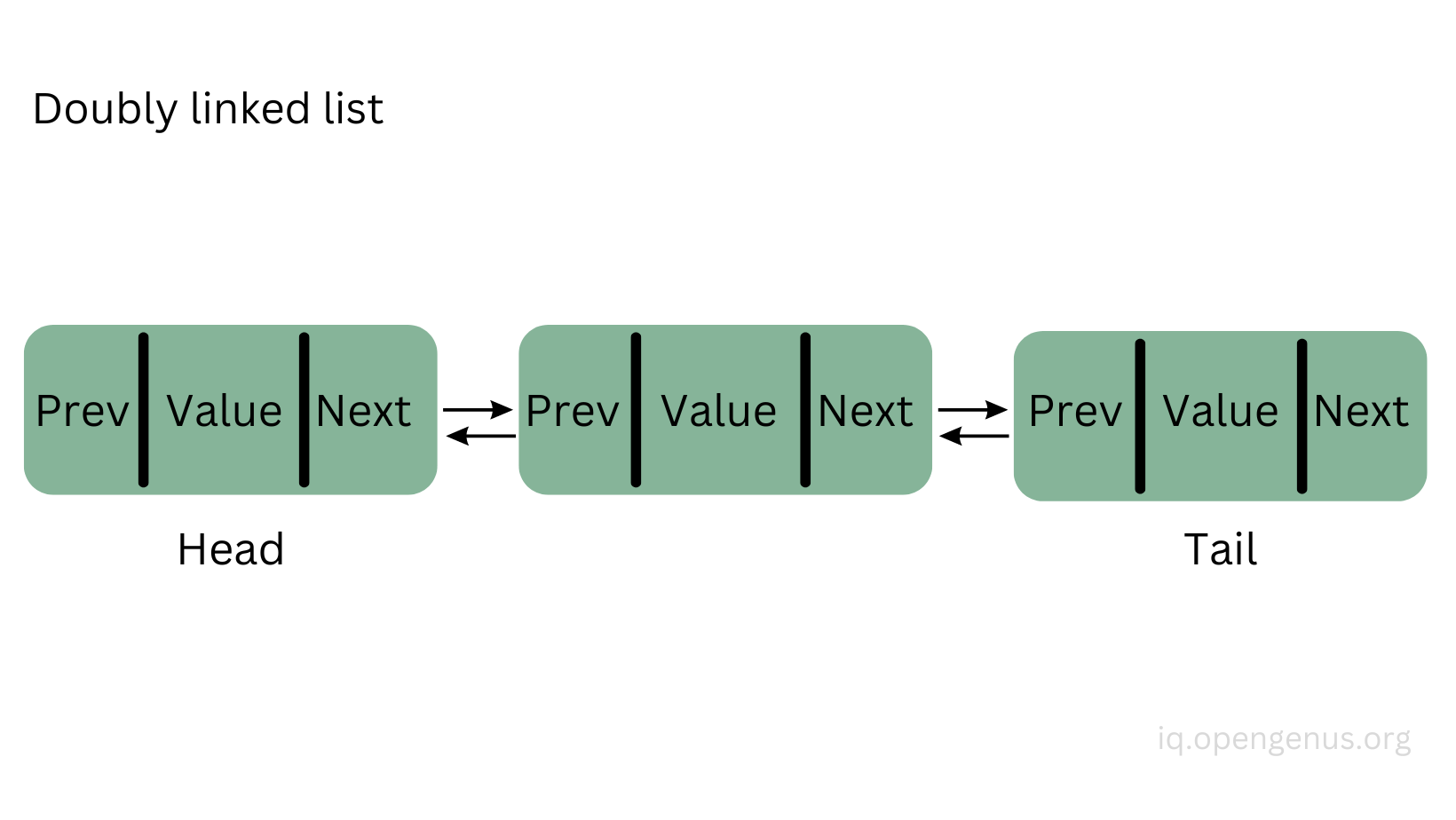In the doubly linked lists the nodes have three properties, previous, value, and next. So here, we can traverse the list in both directions, the next property of the node will point to the next node in the list and the previous property of the node will point back to the previous node in the list. Notice here that head.previous and tail.next will point to null since they are the first and the last nodes in the list. Doubly linked lists are more flexible to work with than singly linked lists.

Let us see how we can create doubly linked lists and some of the methods we can use on them.

## 2. Methods

We need to know how to create a list first and then we need to to be able to create nodes as well in the list. An easy way to set things up is by using classes.

``````class DoublyLinkedList {
constructor() {
this.tail = null;
this.length = 0;
}
}
``````

We create a class called DoublyLinkedList (you can call it anything but this name is suitable) and inside the constructor we assign this.head and this.tail to point to null and the length of the list will start from 0. So when we first create a list we don't have any nodes yet so the head and the tail will point to null.
Let's create our list:

``````const doublyLinkedList = new DoublyLinkedList();
``````

Our list is created above and from here we can add/append nodes to it but first let's create a Node class which will be responsible for creating nodes.

``````class Node {
constructor(value) {
this.value = value;
this.previous = null;
this.next = null;
}
}
``````

When we will add new nodes inside the list, we will have to give them a value as well since they have a value property, so we pass in a value parameter in the constructor and assign it to the value property (this.value). Then, we need to assign the previous and the next property to null because at first they will not point to any nodes yet. And that's all this Node class does, just creating new nodes.

To work with the list, wee need to create methods inside the class.

Let's see our first method which appends a new node in the list.

- append()

``````append(value) {
const node = new Node(value);

this.tail = node;
} else {
node.previous = this.tail;
this.tail.next = node;
this.tail = node;
}
this.length++;
}
``````

The append() method will add a new node in the back of the list.

First, we create a new node with the value we pass in as an argument, then we need to check if the list has nodes yet or not.

If this is the first node, then we assign the head and the tail to this node, otherwise, we set the node's previous property to point to the tail, the tail's next property to point to the new node, then the new node should be the tail, and we increment the length by one.

Let's add 2 nodes to our list first:

``````doublyLinkedList.append(3); // null <=> 3 <=> null
doublyLinkedList.append(12); // null <=> 3 <=> 12 <=> null
``````

And then let's create a third node, I provided images to visualize this process:

``````doublyLinkedList.append(5); // null <=> 3 <=> 12 <=> 5 <=> null
``````

Create the new node: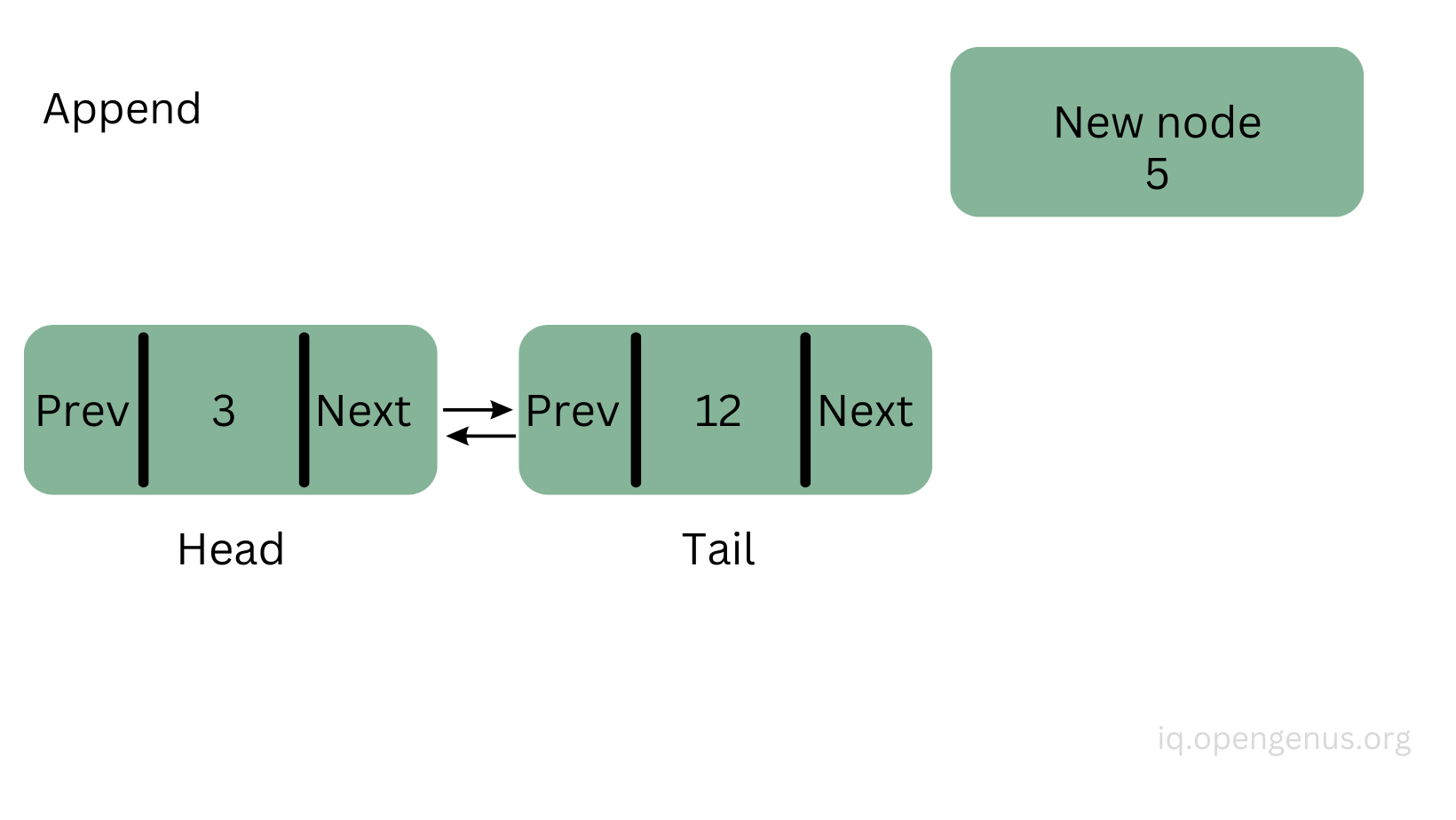Point the node's previous property to the tail: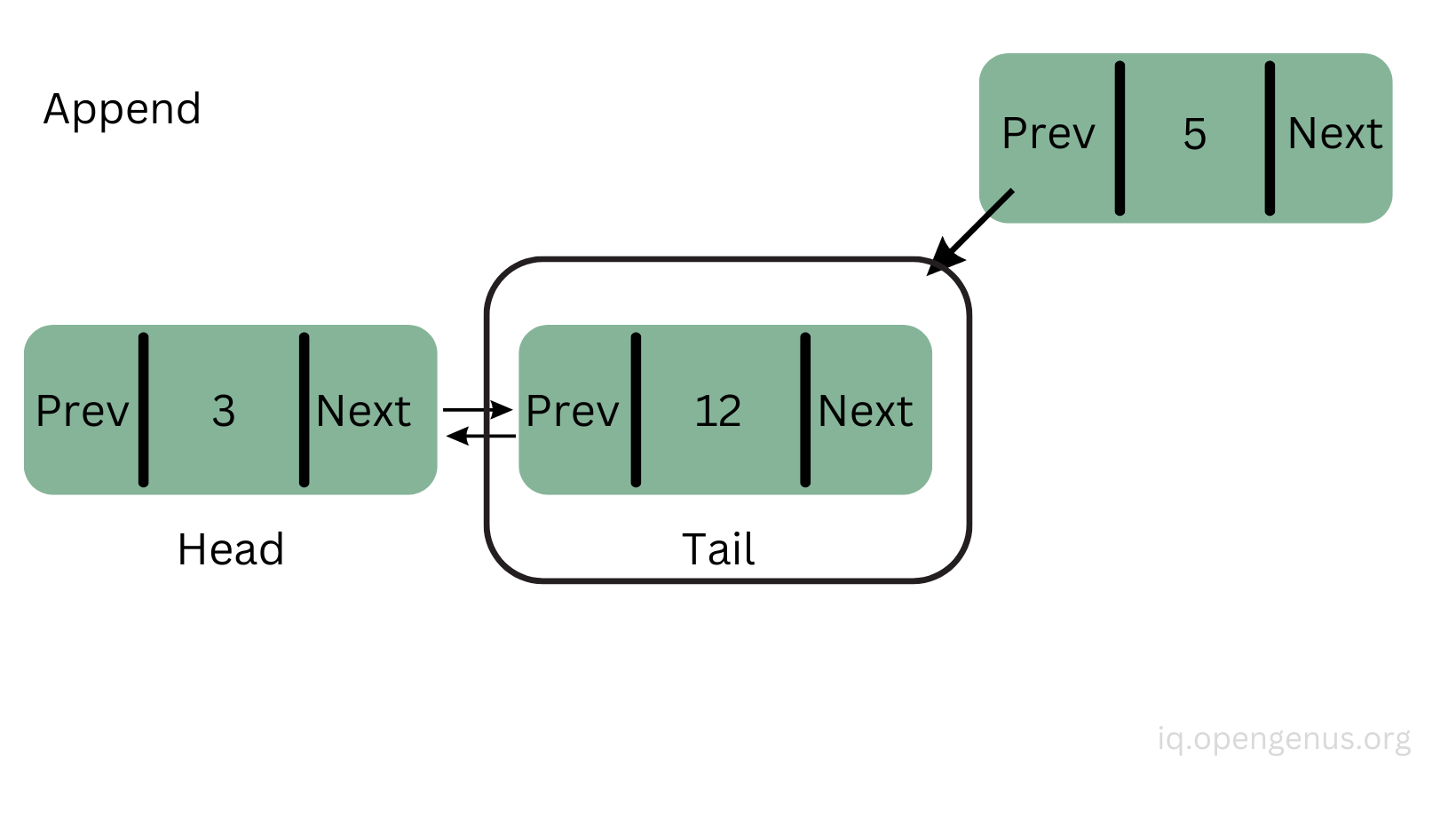Then point the tail's next property to the new node: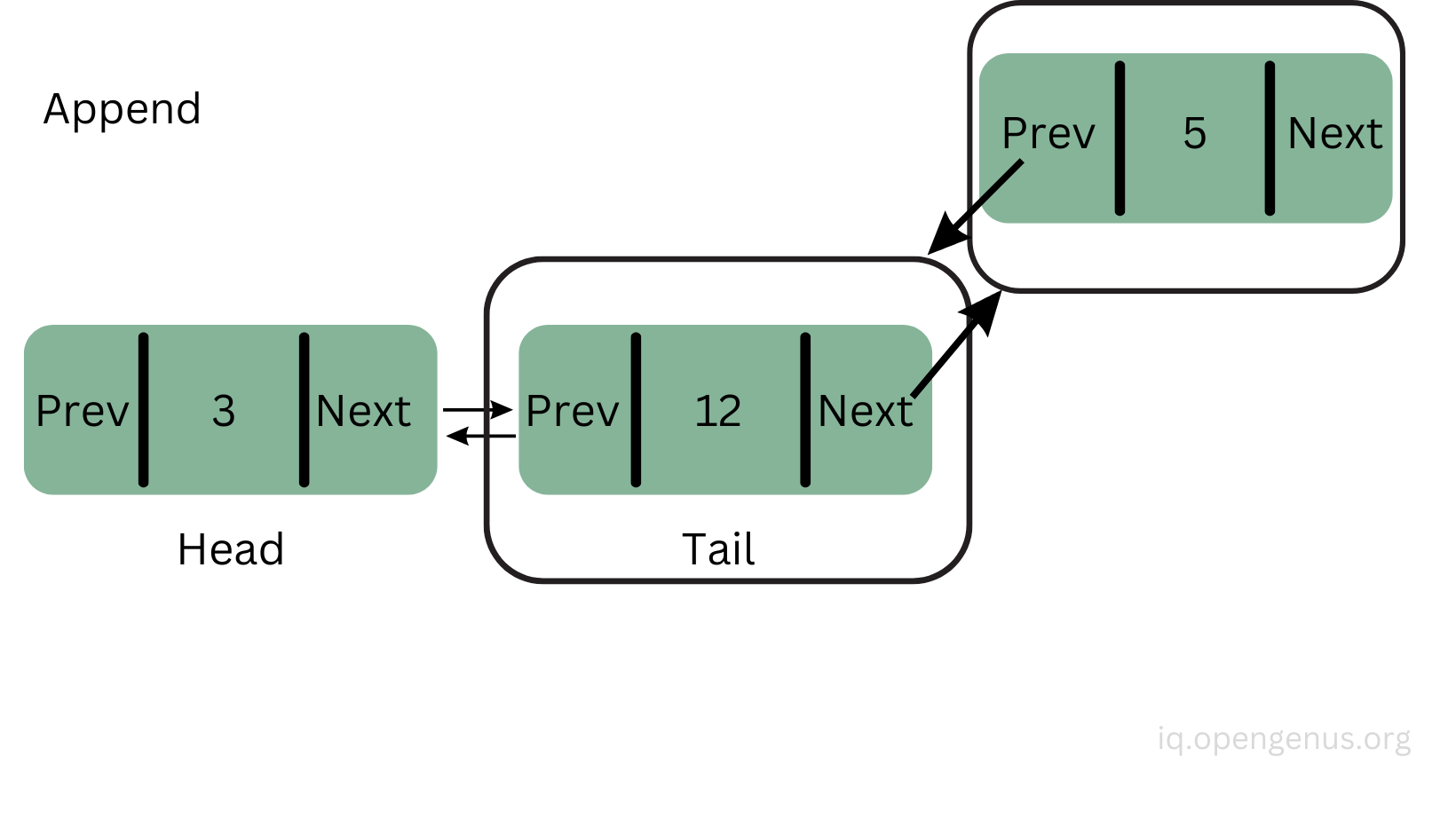Finally, they are connected (linked) to each other so we can set the tail to be the new node: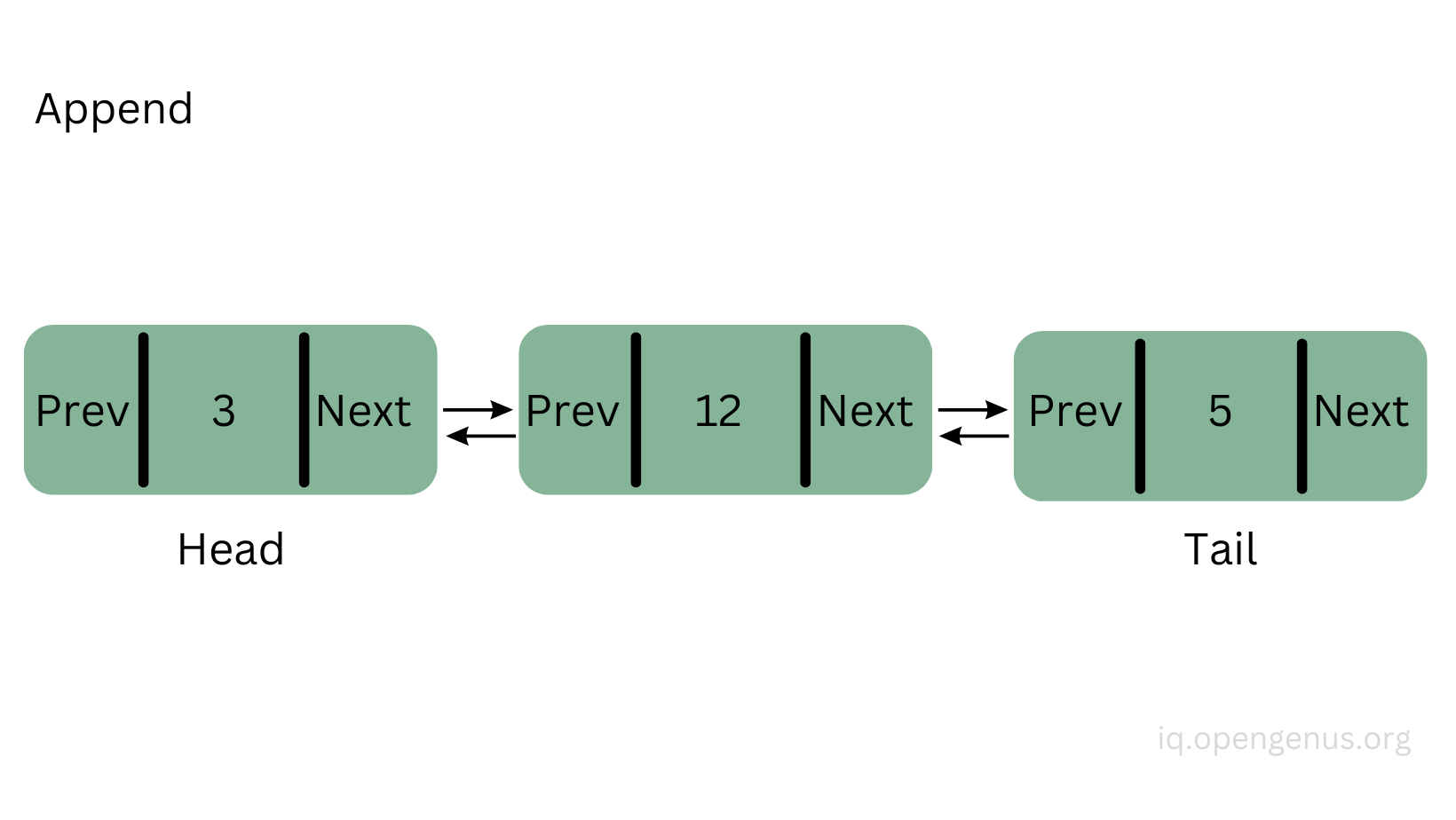- prepend()

``````prepend(value) {
const node = new Node(value);

this.tail = node;
} else {
}
this.length++;
}
``````

The prepend() method is almost the same thing as the append() method, but here we add a new node at the front of the list and the new node will be the head.

``````doublyLinkedList.prepend(1)  // null <=> 1 <=> 3 <=> 12 <=> 5 <=> null
``````

- insertAtIndex(value, index)

``````insertAtIndex(value, index) {
if (index < 0 || index > this.length) {
return;
}

if (index === 0) {
this.prepend(value);
} else if (index === this.length) {
this.append(value);
} else {
const node = new Node(value);
let i = 0;

while (i < index) {
current = current.next;
i++;
}

node.previous = current.previous;
node.next = current;
current.previous.next = node;
current.previous = node;

this.length++;
}
}
``````

If we want to add a new node at a certain index point then we can use the insertAtIndex() method.

First, we check if the index that was passed as the argument is not a negative number and that it's greater than the length of the list. If not, then we don't do anything to the list and we just return.

If the index is 0, that means we want to insert a node at the 0 index which is the first place. So we just call the prepend method and pass the value as well (this.prepend(value)).

If the index is the same as the length of the list, then we need to append the node to the end.

Otherwise, we need to add the node somewhere in between. After we created the new node we set the current node to be the head. We are going to traverse the list from here and go until we reach the index point.

``````let i = 0;

while (i < index) {
current = current.next;
i++;
}
``````

Once we reached the index point, we need to start linking the nodes together.
Let's say we want to insert the newly created node with the value 7 between the node(3) and node(12) which would be at index 2. The current node is the node with the value 12.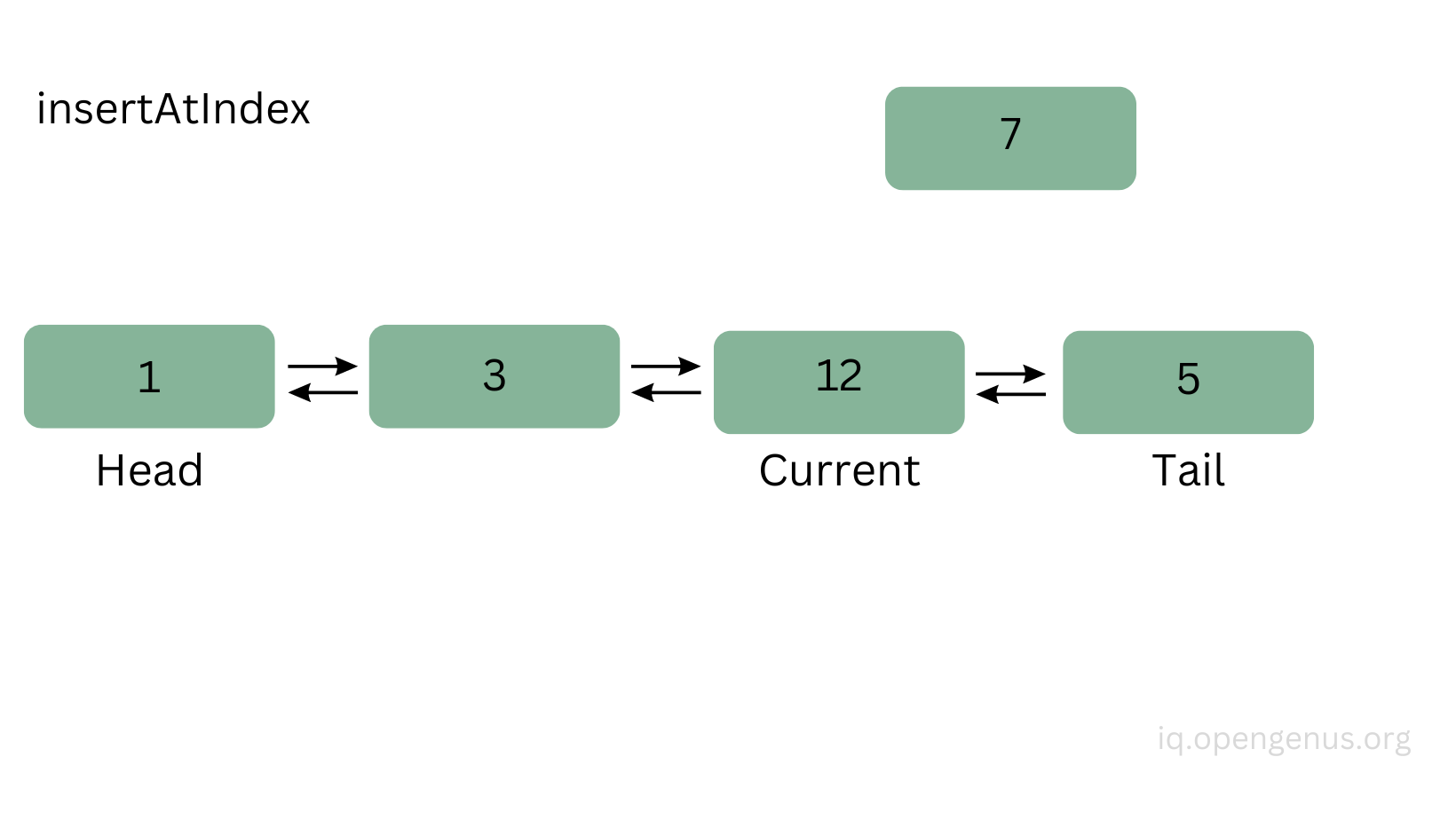We set the new node's previous property to point to the current's previous node: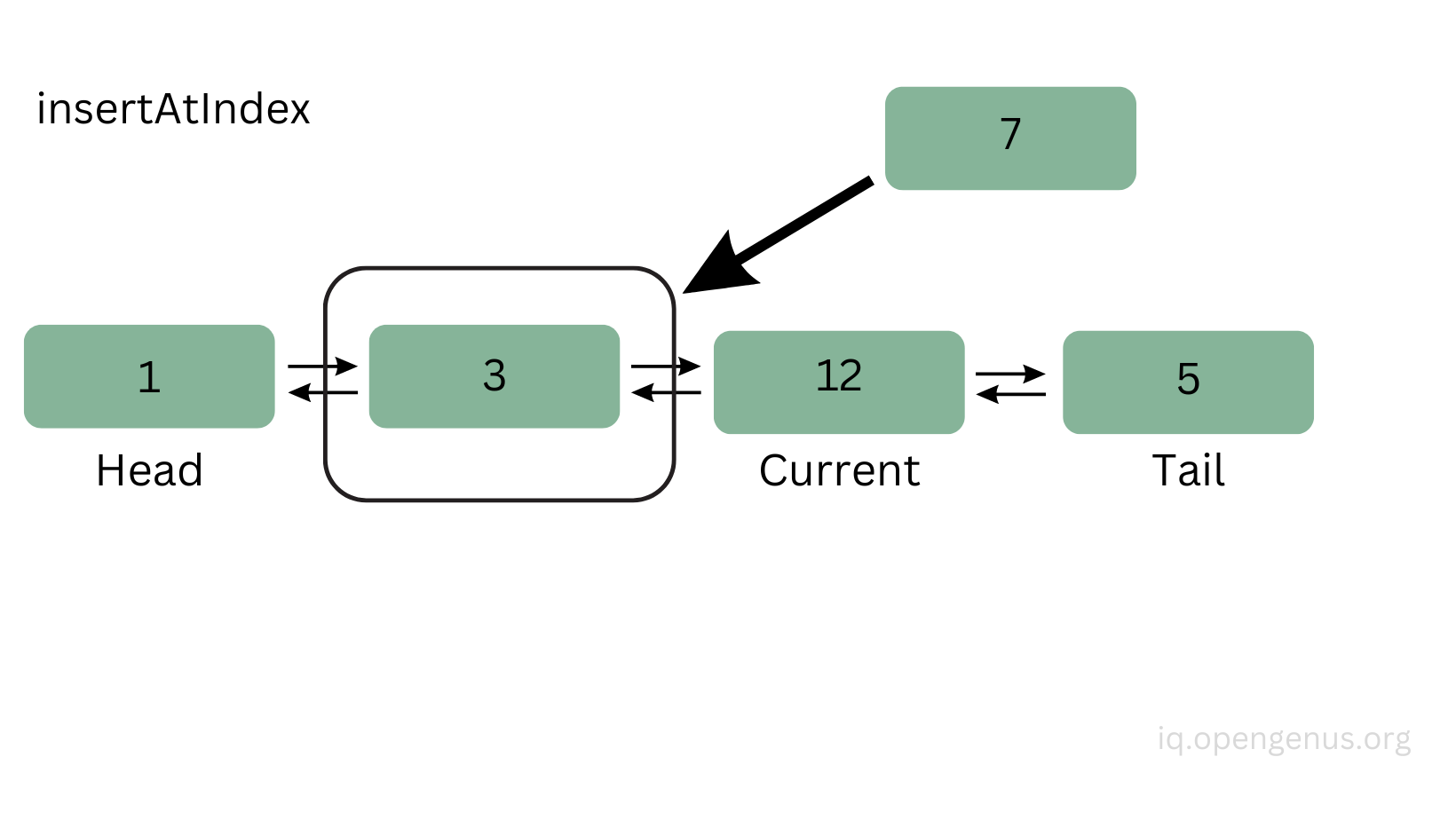Then the new node's next property to point to the current node: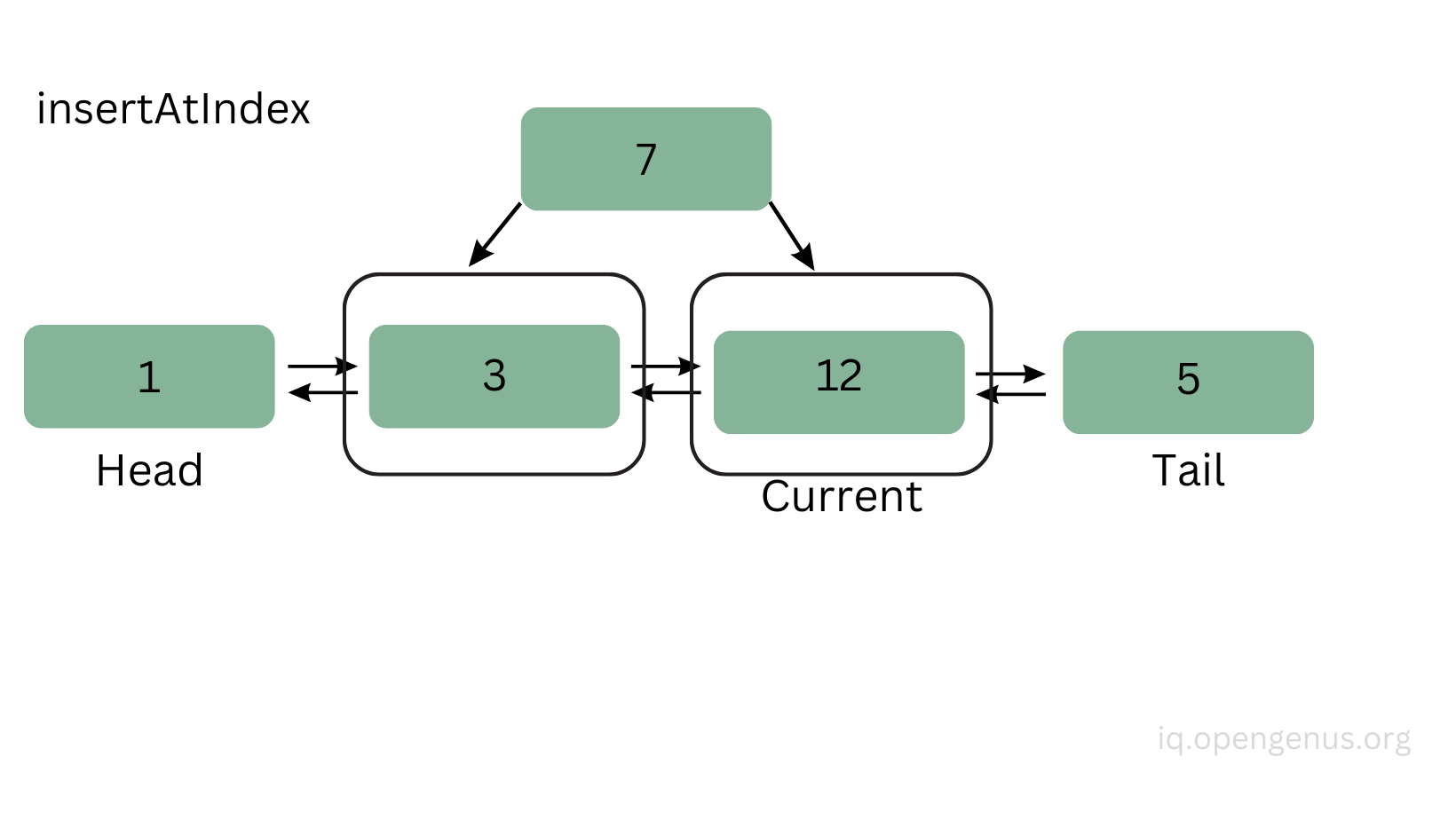Then the next property of the current node's previous node (which is the node with the value 3) to point to the new node (current.previous.next = node):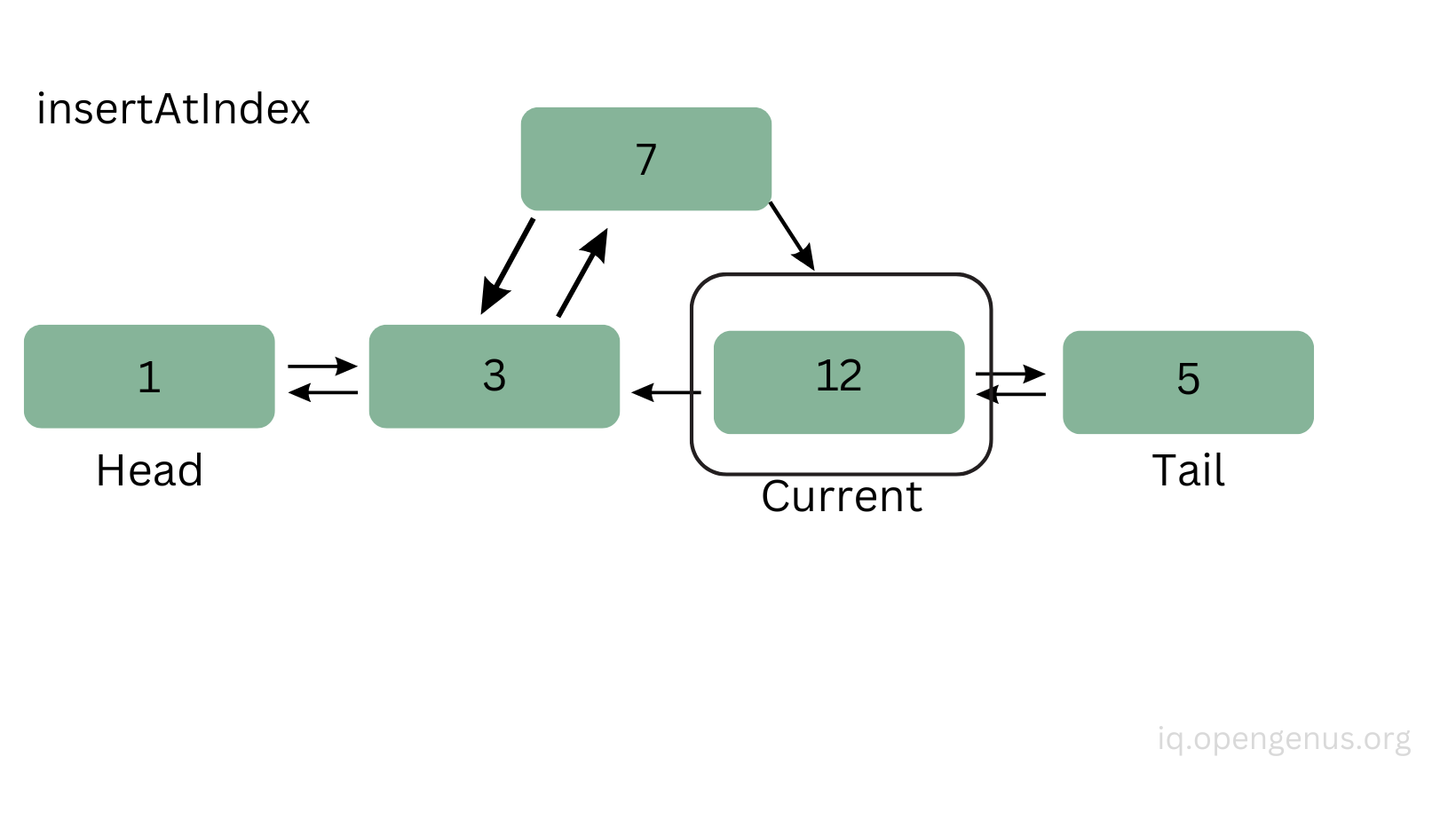Finally, the current node's previous property should point to the new node and increment the length of the list by one. You see that the link/connection between node(3) and node(12) has been removed and added to the new node(7):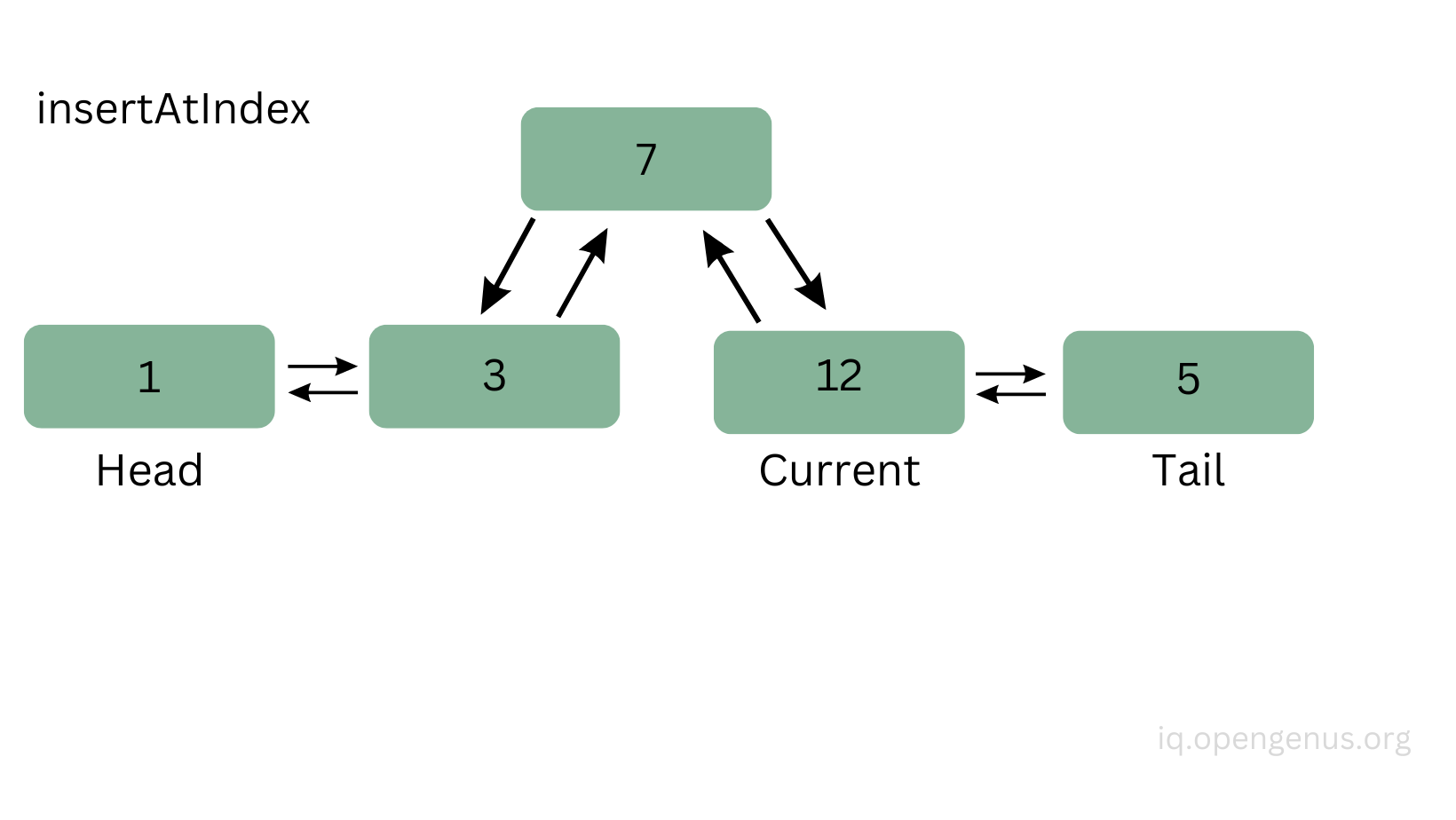``````doublyLinkedList.insertAtIndex(7, 2); // null <=> 1 <=> 3 <=> 7 <=> 12 <=> 5 <=> null
``````

- removeFirst()

`````` removeFirst() {
if (this.length === 0) {
return;
} else if (this.length === 1) {
this.tail = null;
this.length = 0;
} else {
this.length--;
}
}
``````

Now, let's see the methods for removing nodes from the list.

To remove the first node in the list, we can create a method called removeFirst() and start checking if the list has any nodes or if it's empty.
If it's empty, then we don't do anything to the list and we just return.
If the list has only one node, then we remove that node by setting the head and the tail to point to null, and the length to be 0 again.
If it has more nodes, then the head will be the next node (this.head = this.head.next), the head's previous node will point to null, and then we decrement the length of the list by one.

The head will become the second node in the list: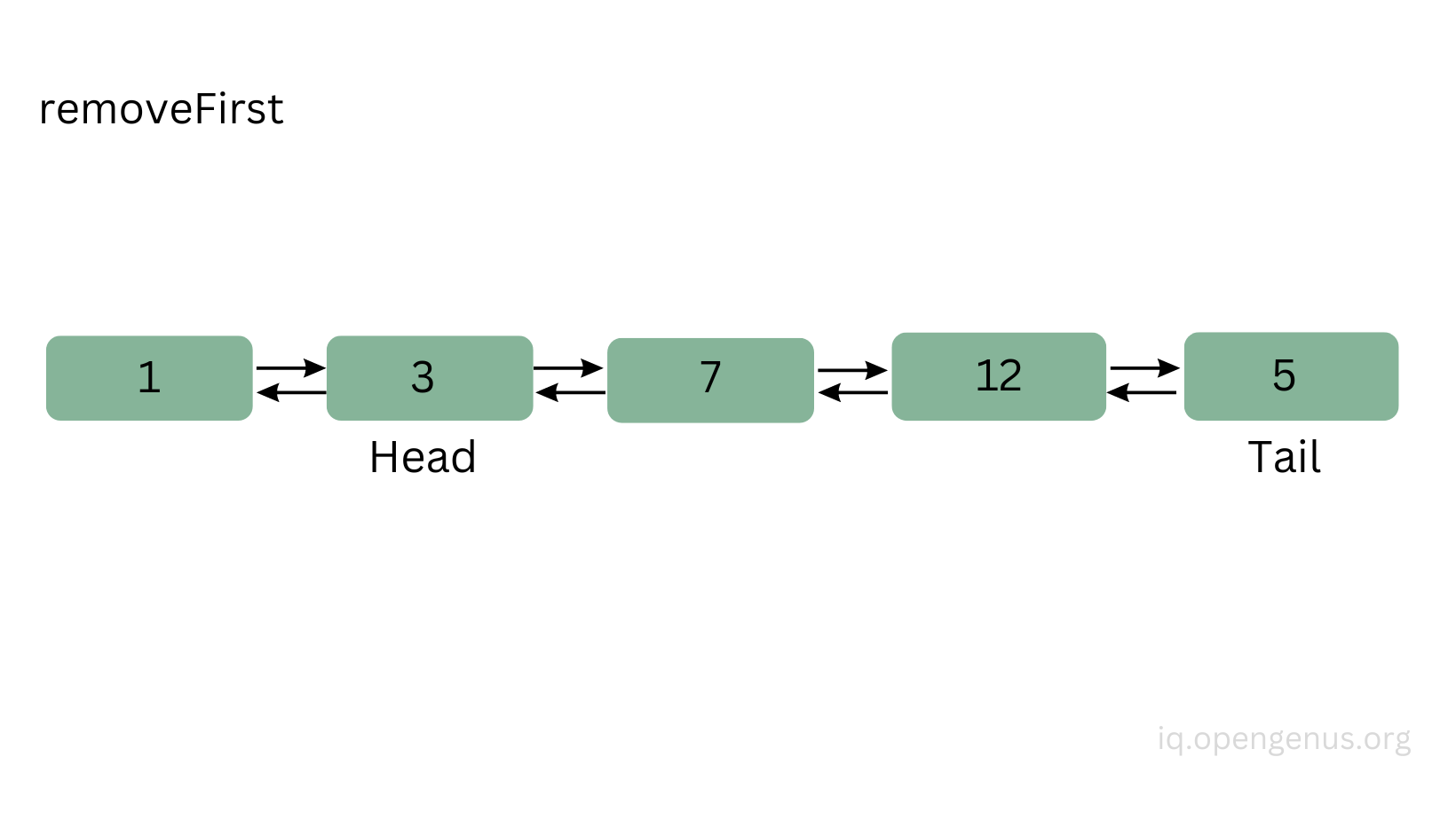Then the previous property of the new head will point to null: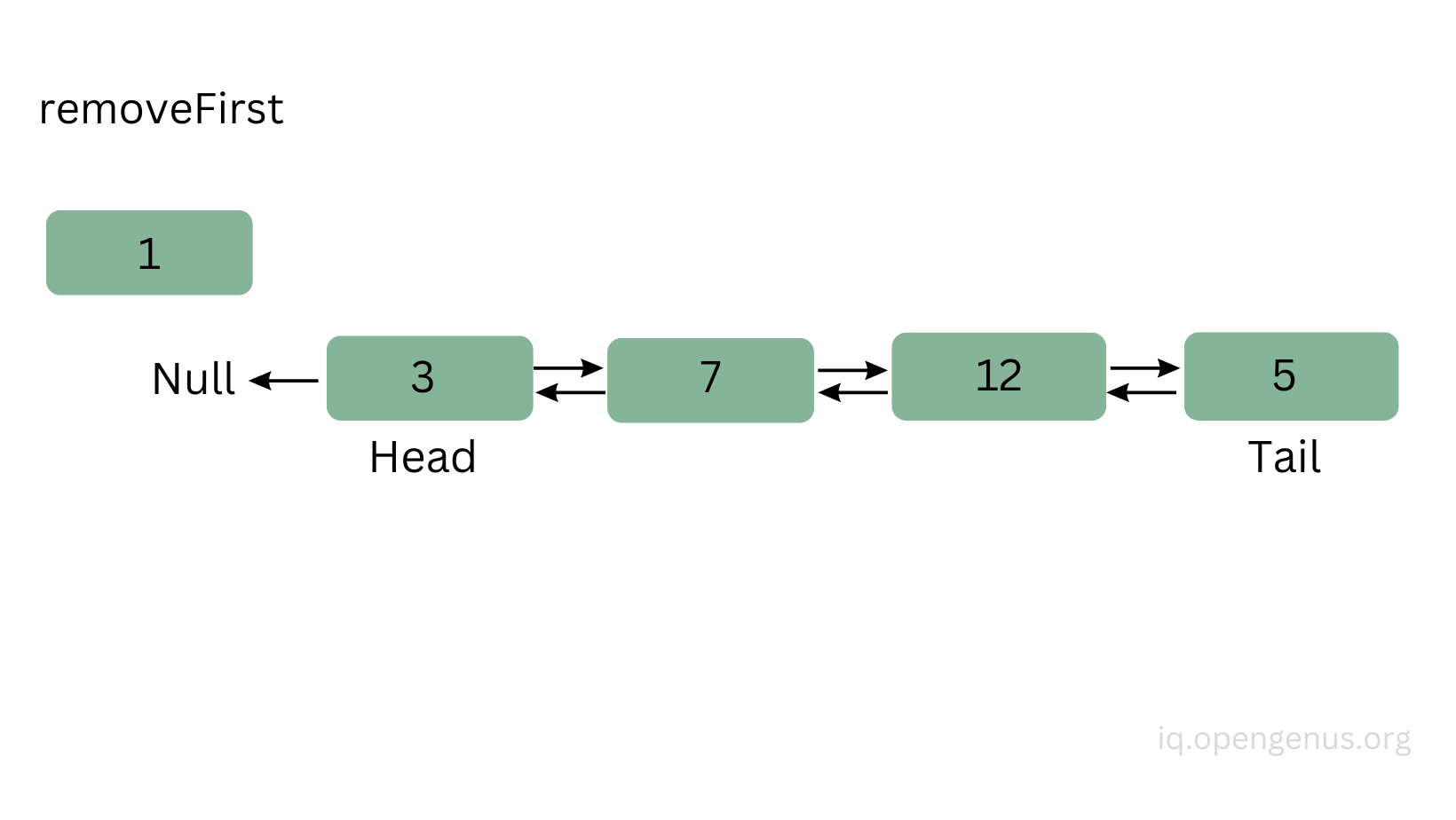``````doublyLinkedList.removeFirst(); // null <=> 3 <=> 7 <=> 12 <=> 5 <=> null
``````

With this, we just removed the node with the value 1.
- removeLast()

`````` removeLast() {
if (this.length === 0) {
return;
} else if (this.length === 1) {
this.tail = null;
this.length = 0;
} else {
this.tail = this.tail.previous;
this.tail.next = null;
this.length--;
}
}
``````

To remove the last node in the list is basically the same just here we have to work with the tail. The new tail will become the previous node of the last node, the new tail's next property will point to null, and we decrement the length of the list by one.

``````doublyLinkedList.removeLast(); // null <=> 3 <=> 7 <=> 12 <=> null
``````

With this, we just removed the last node with the value of 5.

- removeAtIndex(index)

`````` removeAtIndex(index) {
if (index < 0) {
return;
}

if (index === 0) {
this.removeFirst();
} else if (index >= this.length) {
this.removeLast();
} else {
let i = 0;

while (i < index) {
current = current.next;
i++;
}

current.previous.next = current.next;
current.next.previous = current.previous;
this.length--;
}
}
``````

Let's see a method when we want to remove a node somewhere between the first and the last node.
First, we check if the index is a negative number, if it is, then we don't do anything to the list and return.
If the index is 0, then we should remove the first node, so we can just call the removeFirst() method.
If the index is greater or equal than the length of the list, then we should remove the last node, so we can just call the removeLast() method.
Otherwise, we need to traverse the list from a starting point (which is the head) and go until we reach the index point. Once we reached the index point, we need to remove the links/connections between the node we want to remove.
Let's say we want to remove the second node in the list:
The current node will be node(7) because this is where we stopped when traversing the list:

``````let current = this.head;
let i = 0;

while (i < index) {
current = current.next;
i++;
}
``````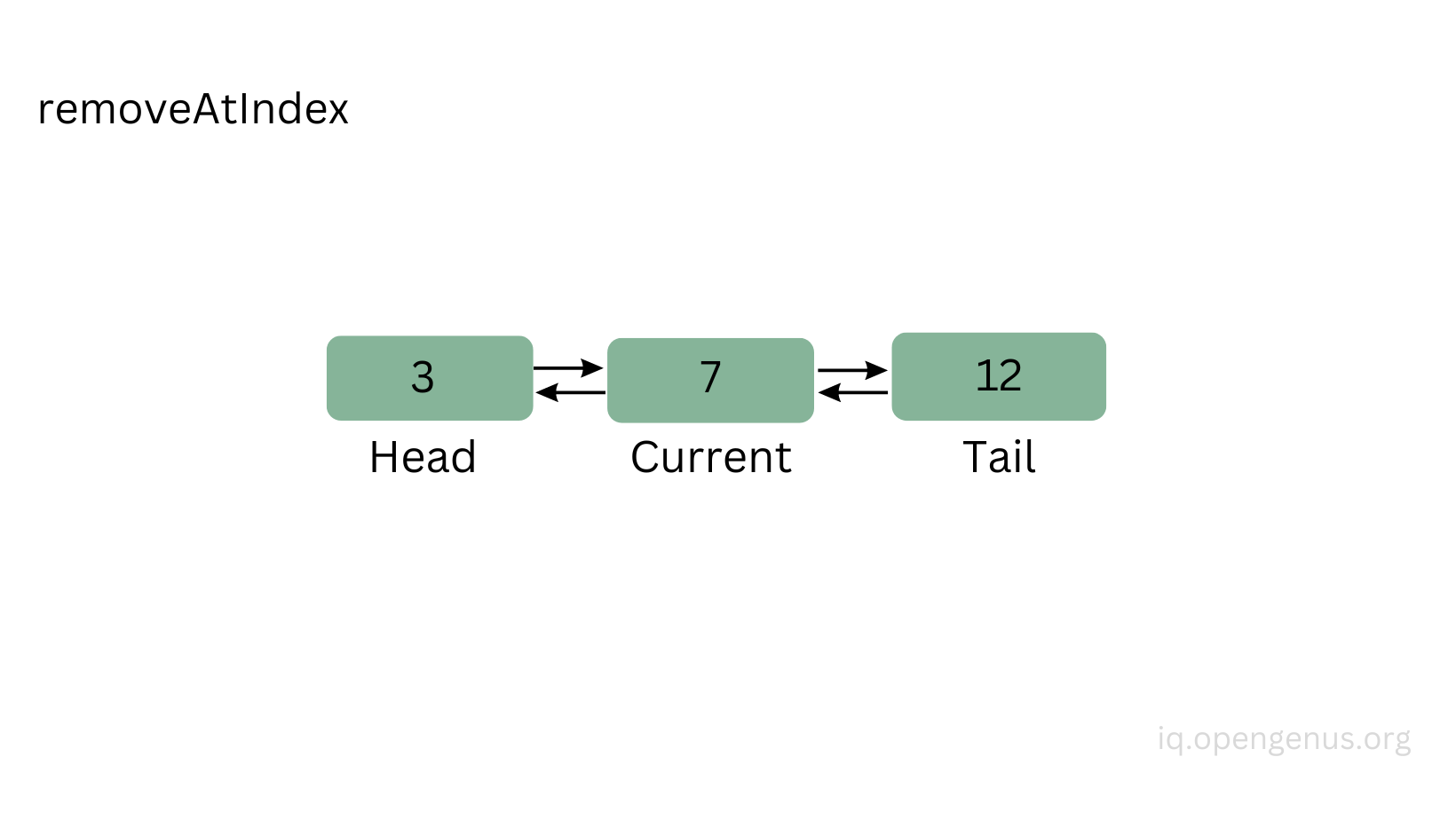Then the next property of the current node's previous node (node(3)) will point to the current's node next node (node(12)):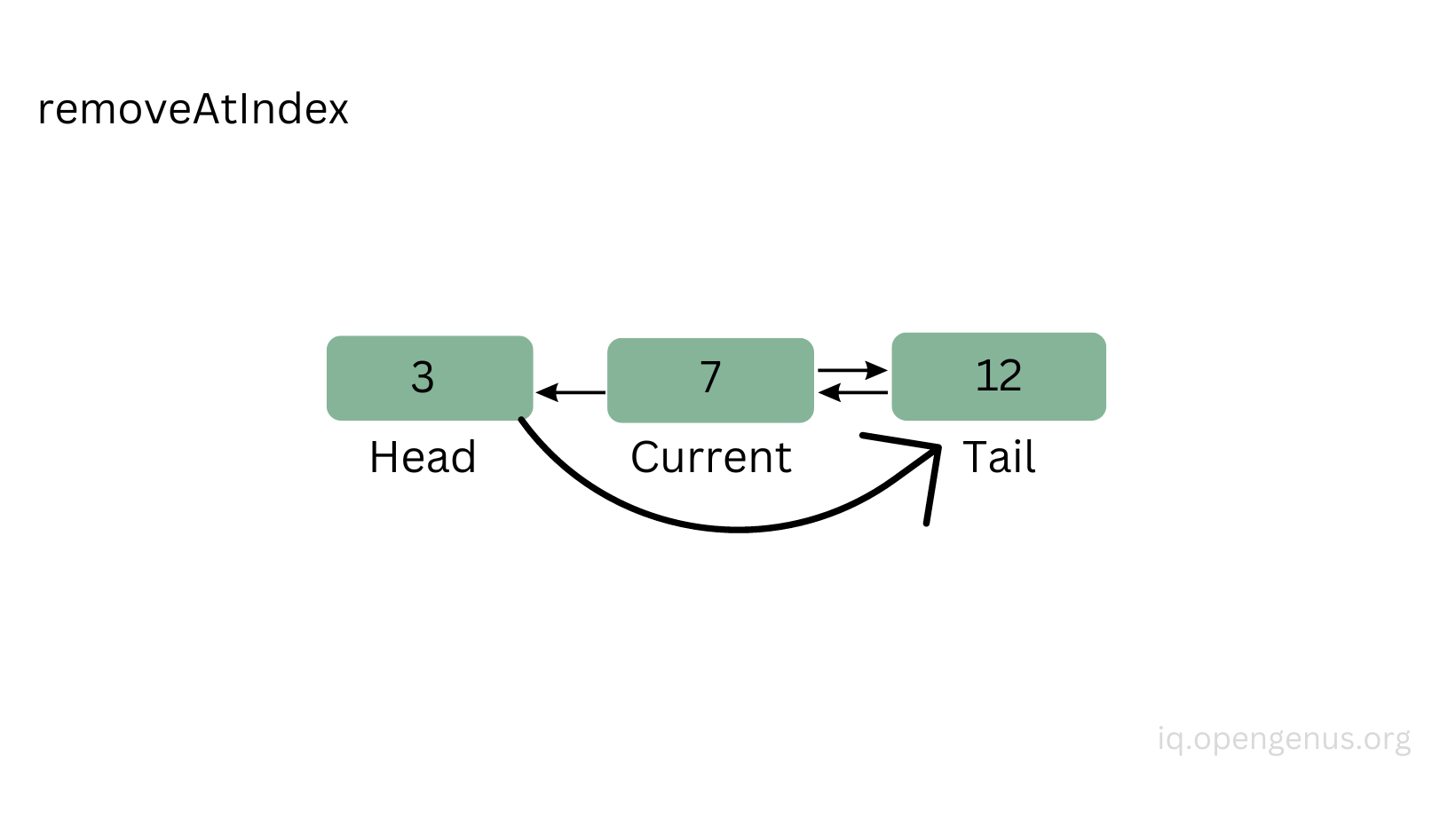Finally, the previous property of the current node's next node (node(12)) will point to the current's node previous node (node(3)), and we will decrement the length of the list by one: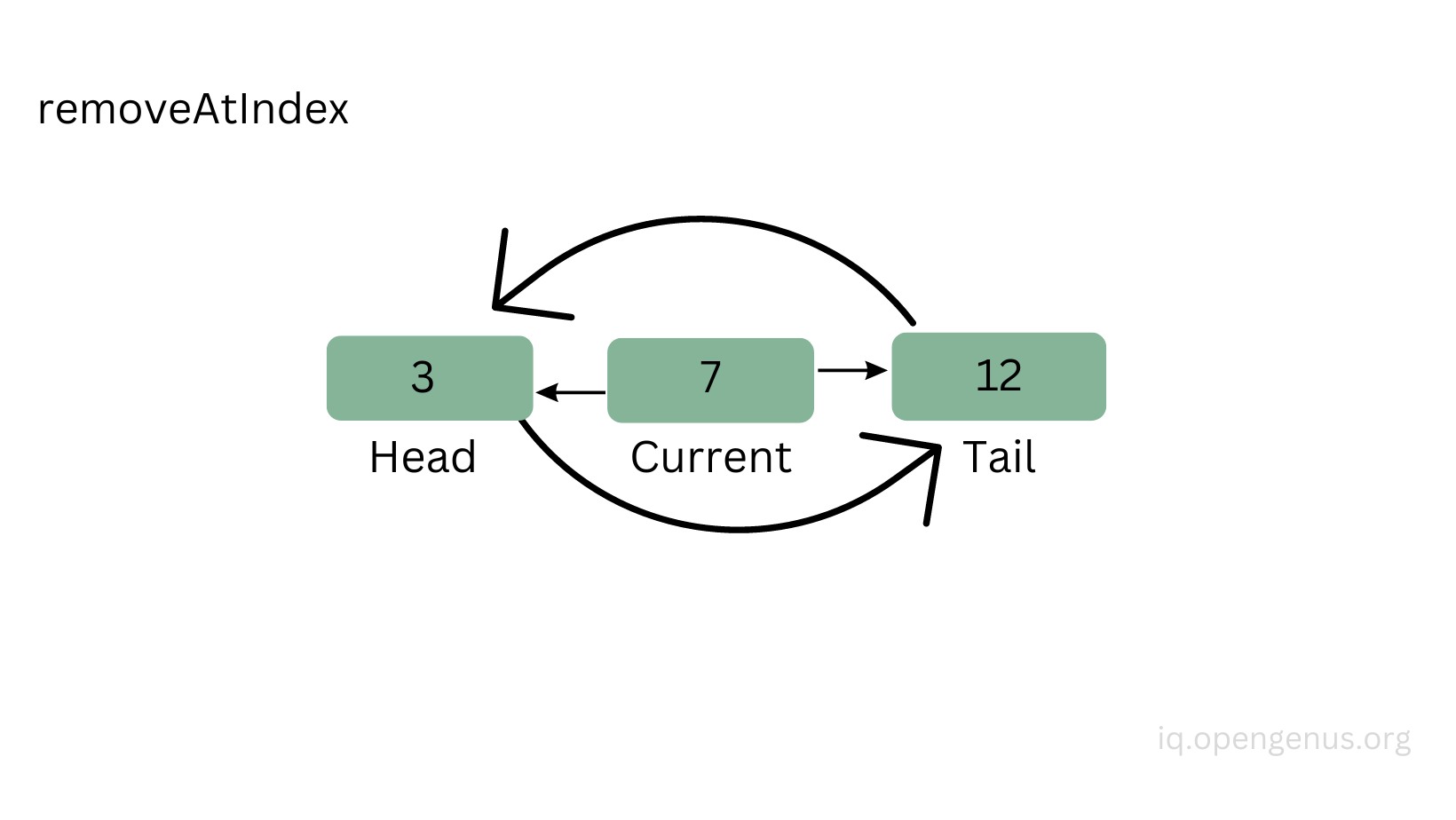``````doublyLinkedList.removeAtIndex(1) // null <=> 3 <=> 12 <=> null
``````

- getValueAtIndex(index)

`````` getValueAtIndex(index) {
if (index < 0 || index >= this.length) {
return;
}
let i = 0;

while (i < index) {
current = current.next;
i++;
}
return current.value;
}
}
``````

The last method I will show you is to get/read a value at a specific index point.
If the index is a negative number or if it's equal or greater than the length of the list, then we don't do anything to the list and just return.
Otherwise, we will traverse the list from a starting point (which is the head) and go until we reach the index point and return the value of the current node.

``````doublyLinkedList.getValueAtIndex(1) // 12
``````

## 3. Summary

Some use cases of linked lists:

• when clicking the arrow buttons in the browser tab to see the previous history (previous page) for example
• or in a music app the previous and forward buttons go to the next song in the playlist

Overall, linked lists are a great way to store or structure data when it fits the application requirements. You can choose to work with either singly or doubly linked lists, keep in mind that doubly lists are more flexible but they require more memory because each node has two references instead of just one.

## Complete JavaScript Implementation

The code might look a bit long but it's not complicated at all to understand it, here's the link to the repository: Doubly linked list

Following is the complete JavaScript implementation of Doubly Linked List

``````// By default each new node will have a value and the previous and next will point to null.
class Node {
constructor(value) {
this.value = value;
this.previous = null;
this.next = null;
}
}

// When the list will be created at the first time, the head and tail will be null (because there are no nodes yet) and the length will be set to 0 as well.
constructor() {
this.tail = null;
this.length = 0;
}

append(value) {
const node = new Node(value);

// If there are no nodes yet in the list, meaning there's no head in the list yet, then we assign the new node to be the head and the tail
this.tail = node;
} else {
// If there are nodes in the list, then we point the node.previous to the current tail and then we point the current.tail.next to the new node and finally we will point the current tail to the new node, so the new node will become the tail.
node.previous = this.tail;
this.tail.next = node;
this.tail = node;
}
this.length++;
}

prepend(value) {
const node = new Node(value);

// If there are no nodes yet in the list, meaning there's no head in the list yet, then we assign the new node to be the head and the tail
this.tail = node;
} else {
// If there are nodes in the list, then we point the node.next to the current head and then we point the current.head.previous to the new node and finally we will point the current head to the new node, so the new node will become the head.
}
this.length++;
}

insertAtIndex(value, index) {
// If the index is a negative number or if it's smaller than the length of the list, then we don't do anything and return.
if (index < 0 || index > this.length) {
return;
}
// If the index is 0, then we just prepend the node at the front of the list.
if (index === 0) {
this.prepend(value);
} else if (index === this.length) {
// If the index is the same number as the length of the list, then we just append the node at the end of the list.
this.append(value);
} else {
const node = new Node(value);
// If the index is in between the first and last node, then we will mark the head as the current value and then we will walk in the list node by node until we reach the index point.
let i = 0;

while (i < index) {
current = current.next;
i++;
}

// Once we reached the index point, then we will add the new node between the current.previous and current.
node.previous = current.previous;
node.next = current;
current.previous.next = node;
current.previous = node;

this.length++;
}
}

removeFirst() {
// If the list is empty then don't do anything and just return.
if (this.length === 0) {
return;
} else if (this.length === 1) {
// If the length of the list is 1, then we set the head and the tail to null, removing the only nde in the list.
this.tail = null;
this.length = 0;
} else {
// If there are more nodes in the list, then we set the head to be the this.head.next and set the this.head.previous to be null.
this.length--;
}
}

removeLast() {
// If the list is empty then don't do anything and just return.
if (this.length === 0) {
return;
} else if (this.length === 1) {
// If the length of the list is 1, then we set the head and the tail to null, removing the only nde in the list.
this.tail = null;
this.length = 0;
} else {
// If there are more nodes in the list, then we set the tail to be the this.tail.previous and set the this.tail.next to be null.
this.tail = this.tail.previous;
this.tail.next = null;
this.length--;
}
}

removeAtIndex(index) {
// If the index is a negative number then we don't do anything and return.
if (index < 0) {
return;
}
// If the index is 0 then we need to remove the first node.
if (index === 0) {
this.removeFirst();
} else if (index >= this.length) {
// If the index is greater or equal than the length of the list, then we need to remove the last node.
this.removeLast();
} else {
// If the index is in between the first and last node, then we will mark the head as the current value and then we will walk in the list node by node until we reach the index point.
let i = 0;

while (i < index) {
current = current.next;
i++;
}
// Once we reached the index point, then we need to remove the current node by linking the current.previous and current.next to each other, leaving the current node out.
current.previous.next = current.next;
current.next.previous = current.previous;
this.length--;
}
}

getValueAtIndex(index) {
// If the index is a negative number or if it's greater or equal than the length of the list, then we don't do anything and just return.
if (index < 0 || index >= this.length) {
return;
}
// We will mark the head as the current node and then we will walk through the list node by node until we reach the index point.
let i = 0;

while (i < index) {
current = current.next;
i++;
}
// Once we reached the index point, we just return the value of the node.
return current.value;
}
}

doublyLinkedList.append(5); // null <=> 5 <=> null
doublyLinkedList.append(3); // null <=> 5 <=> 3 <=> null
doublyLinkedList.prepend(1); // null <=> 1 <=> 5 <=> 3 <=> null
doublyLinkedList.insertAtIndex(8, 2); // null <=> 1 <=> 5 <=> 8 <=> 3 <=> null
doublyLinkedList.removeFirst(); // null <=> 5 <=> 8 <=> 3 <=> null
doublyLinkedList.removeLast(); // null <=> 5 <=> 8 <=> null
doublyLinkedList.prepend(2); // null <=> 2 <=> 5 <=> 8 <=> null
doublyLinkedList.removeAtIndex(5); // null <=> 2 <=> 5 <=> null
doublyLinkedList.removeAtIndex(0); // null <=> 5 <=> null
doublyLinkedList.append(7); // null <=> 5 <=> 7 <=> null
doublyLinkedList.prepend(9); // null <=> 9 <=> 5 <=> 7 <=> null
doublyLinkedList.removeAtIndex(1); // null <=> 9 <=> 7 <=> null